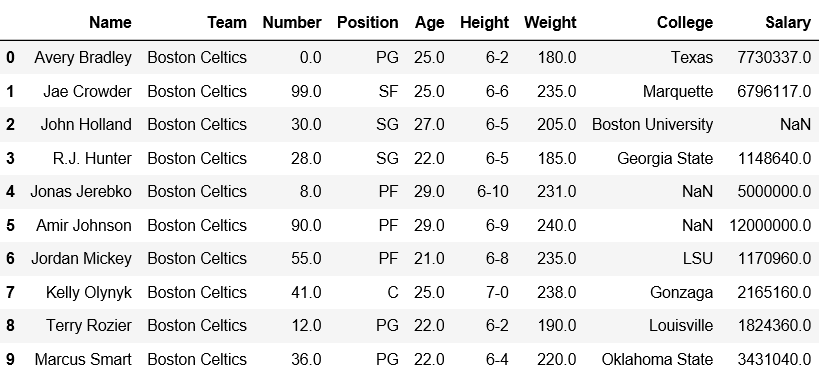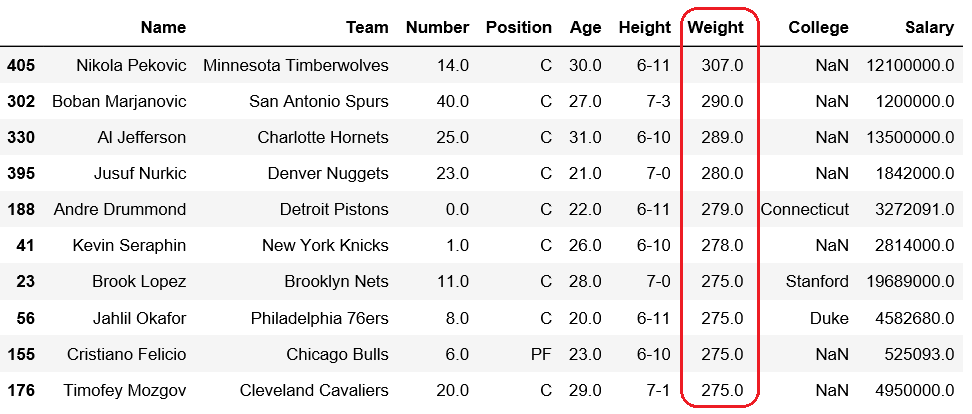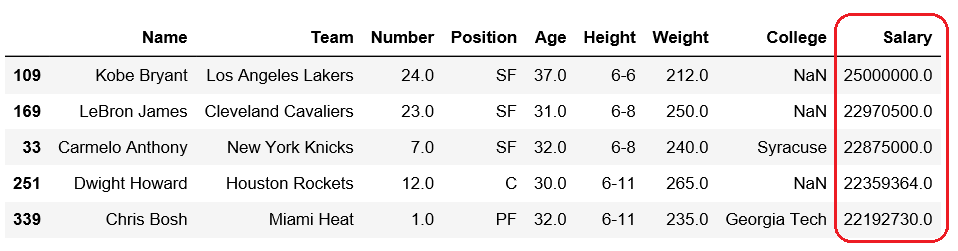GeeksforGeeks App
Open AppBrowser
Continue

# Get n-largest values from a particular column in Pandas DataFrame

Pandas DataFrame is two-dimensional size-mutable, potentially heterogeneous tabular data structure with labeled axes (rows and columns).

Let’s see how can we can get n-largest values from a particular column in Pandas DataFrame.

Observe this dataset first. We’ll use ‘Age’, ‘Weight’ and ‘Salary’ columns of this data in order to get n-largest values from a particular column in Pandas DataFrame.

 `# importing pandas module ``import` `pandas as pd ``   ` `# making data frame ``df ``=` `pd.read_csv(``"https://media.geeksforgeeks.org/wp-content/uploads/nba.csv"``) `` ` `df.head(``10``)`Code #1: Getting 5 largest Age

 `# importing pandas module ``import` `pandas as pd ``   ` `# making data frame ``df ``=` `pd.read_csv(``"nba.csv"``) `` ` `# five largest values in column age``df.nlargest(``5``, [``'Age'``])`

Output:Code #2: Getting 10 maximum weights

 `# importing pandas module ``import` `pandas as pd ``   ` `# making data frame ``df ``=` `pd.read_csv(``"nba.csv"``) `` ` `# Ten largest values in column Weight``df.nlargest(``10``, [``'Weight'``])`

Output:Code #3: Getting 10 maximum salary

 `# importing pandas module ``import` `pandas as pd ``   ` `# making data frame ``df ``=` `pd.read_csv(``"nba.csv"``) `` ` `# five largest values in column Salary``df.nlargest(``5``, [``'Salary'``])`

Output:My Personal Notes arrow_drop_up